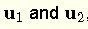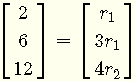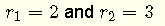# Linear Combinations of Vectors

## Definition of Linear Combinations of Vectors

Vectoris a linear combinations of the vectorsif it can be written in the formwhereare scalars.

## Examples with Solutions

Example 1
Express vectoras a linear combination of the vectorsSolution to Example 1
For vectorto be a linear combination of the vectors, we need to find a scalarssuch that (see definition above)Substituteby their components and scalar multiply to obtain the equationEquality of the components of the vectors above gives the system of equationsThe solutions areHenceExample 2    Show that the vector $$\textbf {v} = \begin{bmatrix} 1 \\ 3 \\ \end{bmatrix}$$ cannot be expressed as a linear combination of the vectors $$\textbf{u}_1 = \begin{bmatrix} 1 \\ 2 \\ \end{bmatrix}$$ and $$\textbf{u}_2 = \begin{bmatrix} 2 \\ 4 \\ \end{bmatrix}$$
Solution to Example 2
We need to show that we cannot find $$r_1$$ and $$r_2$$ such that
$$\begin{bmatrix} 1 \\ 3 \\ \end{bmatrix} = r_1 \begin{bmatrix} 1 \\ 2 \\ \end{bmatrix} + r_2 \begin{bmatrix} 2 \\ 4 \\ \end{bmatrix}$$
Scalar multiply and add the vectors on the right side of the above equation
$$\begin{bmatrix} 1 \\ 3 \\ \end{bmatrix} = \begin{bmatrix} r_1 + 2 r_2 \\ 2 r_1 + 4 r_2\\ \end{bmatrix}$$
Solve the above as a system of equations
$$\begin{array}{lcl} r_1 + 2 r_2 & = & 1 \\ 2 r_1 + 4 r_2 & = & 3 \end{array}$$
Solve the above system using any method. We use the method of elimination.
Multiply the top equation by $$- 2$$
$$\begin{array}{lcl} -2 r_1 - 4 r_2 & = & -2 \\ 2 r_1 + 4 r_2 & = & 3 \end{array}$$
Add the two equations to obtain
$$0 = 1$$
The system has no solution; therefore vector $$\textbf {v}$$ cannot be expressed as a linear combinations of the given vectors $$\textbf {u}_1$$ and $$\textbf {u}_2$$.

Example 3
For what values of $$k$$ the vector $$\textbf {v} = \begin{bmatrix} -1 \\ 5 \\ \end{bmatrix}$$ can be expressed as a linear combination of the vectors $$\textbf{u}_1 = \begin{bmatrix} - 3 \\ 2 \\ \end{bmatrix}$$ and $$\textbf{u}_2 = \begin{bmatrix} k \\ 8 \\ \end{bmatrix}$$
Solution to Example 3
We need to find $$r_1$$ and $$r_2$$ such that
$$\begin{bmatrix} -1 \\ 5 \\ \end{bmatrix} = r_1 \begin{bmatrix} -3 \\ 2 \\ \end{bmatrix} + r_2 \begin{bmatrix} k \\ 8 \\ \end{bmatrix}$$
Scalar multiply and add the vectors on the right side of the above equation
$$\begin{bmatrix} -1 \\ 5 \\ \end{bmatrix} = \begin{bmatrix} -3 r_1 + k r_2 \\ 2 r_1 + 8 r_2\\ \end{bmatrix}$$
Rewrite the above as a system of equations
$$\begin{array}{lcl} -3 r_1 + k r_2 & = & -1 \\ 2 r_1 + 8 r_2 & = & 5 \end{array}$$
For the above system to have solutions $$r_1$$ and $$r_2$$, the determinant.
$$Det \begin{bmatrix} -3 & k \\ 2 & 8 \\ \end{bmatrix} = -24 - 8 k$$
must be different from zero. \)
Solve the inequality $$-24 + 2 k \ne 0$$
to obtain $$k \ne - 12$$
Vector $$\textbf {v}$$ can be expressed as a linear combinations of the given vectors $$\textbf {u}_1$$ and $$\textbf {u}_2$$ for any value of $$k \ne - 12$$.